Courses

# Simple Stresses (Part - 1) Mechanical Engineering Notes | EduRev

## Mechanical Engineering : Simple Stresses (Part - 1) Mechanical Engineering Notes | EduRev

The document Simple Stresses (Part - 1) Mechanical Engineering Notes | EduRev is a part of the Mechanical Engineering Course Machine Design.
All you need of Mechanical Engineering at this link: Mechanical Engineering

Introduction

Stresses are developed in machine elements due to applied load and machine design involves ensuring that the elements can sustain the induced stresses without yielding. Consider a simple lever as shown in figure-2.1.1.1: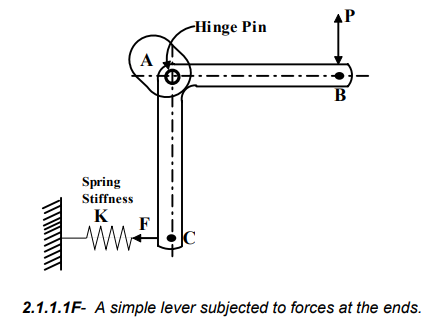A proper design of the spring would ensure the necessary force P at the lever end B. The stresses developed in sections AB and AC would decide the optimum cross-section of the lever provided that the material has been chosen correctly.

The design of the hinge depends on the stresses developed due to the reaction forces at A. A closer look at the arrangement would reveal that the following types of stresses are developed in different elements:

Lever arms AB and AC - Bending stresses

Hinge pin - Shear and bearing stresses.

Spring - Shear stress.

It is therefore important to understand the implications of these and other simple stresses. Although it is more fundamental to consider the state of stress at a point and stress distribution, in elementary design analysis simple average stresses at critical cross-sections are considered to be sufficient.

Some basic issues of simple stresses

Tensile stress

The stress developed in the bar (figure-2.1.2.1) subjected to tensile loading is given by  σ = P/A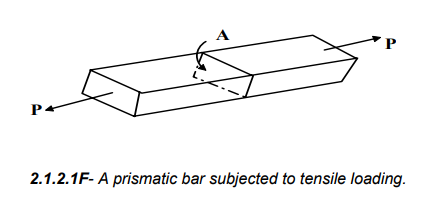Compressive stress

The stress developed in the bar (figure-2.1.2.2) subjected to compressive loading is given by

σc =  P/A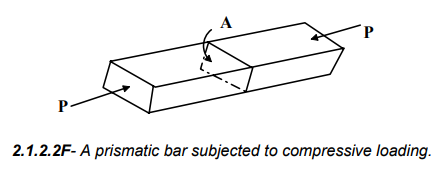Here the force P is the resultant force acting normal to the cross-section A. However, if we consider the stresses on an inclined cross-section B (figure2.1.2.3) then the normal stress perpendicular to the section is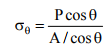and shear stress parallel to the section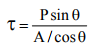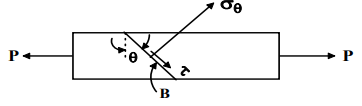2.1.2.3F- Stresses developed at an inclined section of a bar subjected to tensile loading

Bearing stress

When a body is pressed against another, the compressive stress developed is termed bearing stress. For example, bearing stress developed at the contact between a pillar and ground (figure- 2.1.2.4a) is  σbr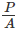at the contact surface between a pin and a member with a circular hole (figure- 2.1.2.4b) is σbr =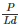and at the faces of a rectangular key fixing a gear hub on a shaft (figure- 2.1.2.4c) is σbr =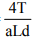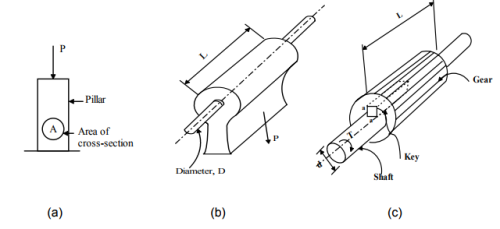2.1.2.4F- The bearing stresses developed in pillar and machine parts.

The pressure developed may be irregular in the above examples but the expressions give the average values of the stresses.

Shear stress

When forces are transmitted from one part of a body to other, the stresses developed in a plane parallel to the applied force are the shear stresses (figure2.1.2.5) and the average values of the shear stresses are given by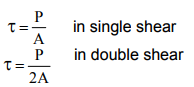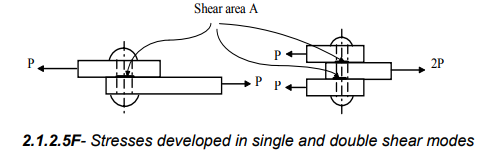In design problems, critical sections must be considered to find normal or shear stresses. We consider a plate with holes under a tensile load (figure-2.1.2.6) to explain the concept of critical sections.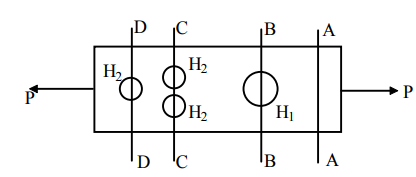2.1.2.6F- The concept of critical sections explained with the help of a loaded plate with holes at selected locations.

Let the cross-sectional area of the plate, the larger hole H1 and the smaller holes H2 be A, a1, a2 respectively. If 2a2 > a1 the critical section in the above example is C and the average normal stress at the critical section is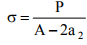Offer running on EduRev: Apply code STAYHOME200 to get INR 200 off on our premium plan EduRev Infinity!

## Machine Design

57 videos|71 docs|102 tests

,

,

,

,

,

,

,

,

,

,

,

,

,

,

,

,

,

,

,

,

,

;